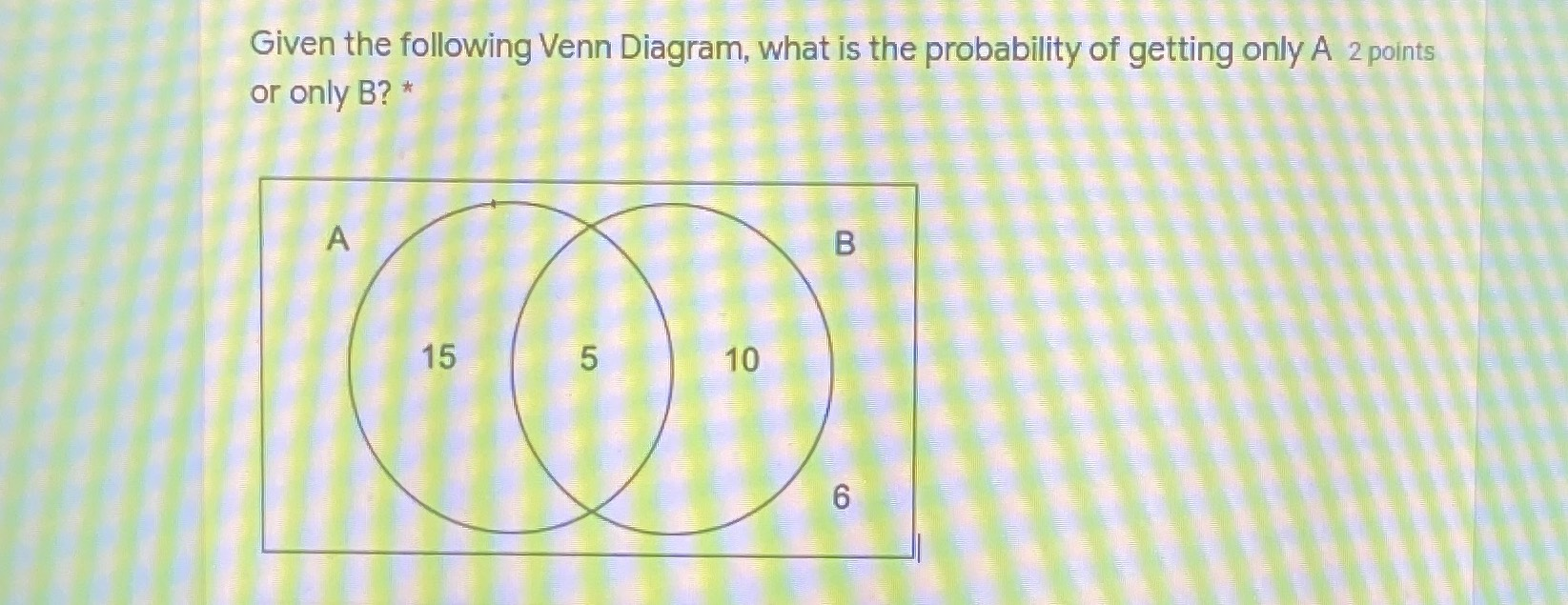### Still have math questions?

Algebra
Question

0Probability of getting only A is $$\frac{15}{36}$$=$$\frac{5}{12}$$